• 参数估计两种形式：点估计与区间估计。本文选择几种常用点估计方法作一些讨论。 用于估计未知参数统计量称为点估计（量）。参数 θθ\theta 估计量常用 θ^=θ^(x1,x2,…,xn)θ^=θ^(x1,x2,…,xn)\hat{\...
参数估计有两种形式：点估计与区间估计。本文选择几种常用的点估计方法作一些讨论。
用于估计未知参数的统计量称为点估计（量）。参数 $\theta$ 的估计量常用 $\hat{\theta} = \hat{\theta}(x_{1},x_{2}, \dots, x_{n})$ 表示，参数 $\theta$ 的可能取值范围称为参数空间，记为 $\Theta = \{\theta\}$。
文章目录最大似然估计贝叶斯估计
最大似然估计
最大似然估计，即对似然函数最大化，其关键是从样本 $x$ 和含有位置参数 $\theta$ 的分布 $p(x,\theta)$ 获得似然函数。设 $x=(x_{1},x_{2},\dots,x_{n})$ 是来自含有未知参数的某分布 $p(x,\theta)$ 的一个样本，那么其联合分布为：
$p(x,\theta) = \prod_{i=1}^{n}p(x_{i},\theta)$ 其中 $p(x_{i},\theta)$ 在连续场合是指密度函数在 $x_{i}$ 处的值，在离散场合为分布列中的一个概率 $P_{\theta}(X=x_{i})$ 。对样本分布 $p(x,\theta)$ 我们知道：

样本如何产生？先有 $\theta$ 后有 $x$，即先有一个给定的 $\theta$ 的值 $\theta_{0}$，然后由分布 $p(x,\theta_{0})$ 经过随机抽样产生样本观察值 $x$。
如今我们有了 $x$ 如何追溯参数 $\theta_{0}$ 呢？当给定样本观察值 $x$ 时样本分布 $p(x,\theta)$ 仅是 $\theta$ 的函数，可记为 $L(\theta,x)$ 或 $L(\theta)$，并称其为似然函数。对于不同的 $\theta_{1},\theta_{2}\in\Theta$，可使得样本观察值 $x$ 出现的机会不同。若 $L(\theta_{1}) > L(\theta_{2})$，表明 $\theta_{1}$ 会使 $x$ 出现的机会比 $\theta_{2}$ 更大些，即 $\theta_{1}$ 比 $\theta_{2}$ 更像真值 $\theta_{0}$。也就是说 $L(\theta)$ 成为了度量 $\theta$ 更像真值的程度，其值越大越像。按此思路，在参数空间 $\Theta$ 中使 $L(\theta)$ 最大的 $\hat{\theta}$ 就是最像 $\theta_{0}$ 的真值，这个 $\hat{\theta}$ 就是 $\theta$ 的最大似然估计。

这里给出两个实例。
1.伯努利分布实例
假设 $P(X=1)=p,P(X=0)=1-p$ 综合起来就有
$P(X)=p^{X}(1-p)^{1-X}$
此时如果有一组数据 $D$ 是从这个随机变量中采样得到的，那么就有
\begin{aligned} \ max_{p}\log P(D)&= \max_{p}\log\prod_{i}^{N}P(D_{i}) \\ &=\max_{p}\sum_{i}^{N}\log P(D_{i}) \\ &=\max_{p}\sum_{i}^{N}[D_{i}\log p+(1-D_{i})\log(1-p)] \end{aligned}
对上式求导，则有
$\nabla_{p}\max_{p}\log P(D)=\sum_{i}^{N}[D_{i}\frac{1}{p}+(1-D_{i})\frac{1}{p-1}]$
求极值，令导数为 $0$，就有
\begin{aligned} & \sum_{i}^{N}[D_{i}\frac{1}{p}+(1-D_{i})\frac{1}{p-1}]=0 \\ & \sum_{i}^{N}[D_{i}(p-1)+(1-D_{i})p]=0 \\ & \sum_{i}^{N}(p-D_{i})=0 \\ & p=\frac{1}{N}\sum_{i}^{N}D_{i} \end{aligned}
即全部采样的平均值。
2.高斯分布实例
令 $p(x)=\frac{1}{\sqrt{2\pi\sigma^{2}}}e^{-\frac{(x-\mu)^{2}}{2\sigma^{2}}}$，采用同样的方法有
\begin{aligned} \max_{p}\log P(D) &= \max_{p}\log\prod_{i}^{N}P(D_{i}) \\ &= \max_{p}\sum_{i}^{N}\log P(D_{i}) \\ &= \max_{p}\sum_{i}^{N}[-\frac{1}{2}\log(2\pi\sigma^{2})-\frac{(D_{i}-\mu)^{2}}{2\sigma^{2}}] \\ &= \max[-\frac{N}{2}\log(2\pi\sigma^{2})-\frac{1}{2\sigma^{2}}\sum_{i}^{N}(D_{i}-\mu)^{2}] \end{aligned}
此处包含两个参数，分别估计。
首先对 $\mu$ 求导，有
$\frac{\partial\max_{\mu}\log P(D)}{\partial \mu} = -\frac{1}{\sigma^{2}}\sum_{i}^{N}(\mu-D_{i})$
令导数为 $0$，有
$-\frac{1}{\sigma^{2}}\sum_{i}^{N}(\mu-D_{i})=0,\quad \mu=\frac{1}{N}\sum_{i}^{N}D_{i}$
注意很容易看出这个结果与最小二乘计算完全相同，实质上最小二乘法可以与极大似然法中假定误差遵循正态分布的特殊情况相对应。
其次对 $\sigma^{2}$ 求导，有
$\frac{\partial\max_{\sigma^{2}}\log P(D)}{\partial\sigma^{2}} = -\frac{N}{2\sigma^{2}}+\frac{1}{2\sigma^{4}}\sum_{i}^{N}(D_{i}-\mu)^{2}$
令导数为 $0$，有
$-\frac{N}{2\sigma^{2}}+\frac{1}{4\sigma^{4}}\sum_{i}^{N}(D_{i}-\mu)^{2}=0$
$\sigma^{2} = \frac{1}{N}\sum_{i}^{N}(D_{i}-\mu)^{2}$
可见最终计算结果与期望方差计算方式完全一致。注意最大似然估计并不一定具有无偏性。
对似然函数添加或剔除一个与参数 $\theta$ 无关的量 $c(x)>0$，不影响寻求最大似然估计的最终结果，故 $c(x)L(\theta)$ 仍然是 $\theta$ 的似然函数。例如，对于正态分布而言：
$L(\mu,\sigma^{2}) = \prod_{i=1}^{n}\frac{1}{\sqrt{2\pi}\sigma^{2}}e^{-\frac{(x_{i}-\mu)^{2}}{2\sigma^{2}}} \propto (\sigma^{2})^{-\frac{n}{2}}\exp\left\{-\frac{1}{2\sigma^{2}}\sum_{i=1}^{n}(x_{i}-\mu)^{2}\right\}$
不变原理： 设 $X\sim p(x,\theta), \theta\in\Theta$，若 $\theta$ 的最大似然估计为 $\hat{\theta}$ ，则对任意函数 $\gamma=g(\theta)$，$\gamma$ 的最大似然估计为 $\hat{\gamma}=g(\hat{\theta})$。
贝叶斯估计
统计学中有两个主要学派：频率学派（又称经典学派）和贝叶斯学派。前述最大似然估计属于经典统计学范畴。频率学派利用总体信息和样本信息进行统计推断，贝叶斯学派与之的区别在于还用到了先验信息。
贝叶斯学派最基本的观点是：任一未知量 $\theta$ 都可以看做随机变量，可用一个概率分布区描述，这个分布称为先验分布 （记为 $\pi(\theta)$）。因为任一未知量都有不确定性，而在表述不确定性地程度时，概率与概率分布是最好的语言。依赖于参数 $\theta$ 的密度函数在经典统计学中记为 $p(x,\theta)$，它表示参数空间 $\Theta$ 中不同的 $\theta$ 对应不同的分布。在贝叶斯统计中应记为 $p(x|\theta)$ ，表示随机变量 $\theta$ 给定某个值时，$X$ 的条件密度函数。
从贝叶斯观点看，样本 $x$ 的产生要分两步进行：首先，设想从先验分布 $\pi(\theta)$ 中产生一个样本 $\theta'$ ，这一步人是看不到的，所以是“设想”；再从 $p(x|\theta')$ 中产生一个样本 $x=(x_{1},x_{2},x_{3},\dots,x_{n})$ 。这时样本 $x$ 的联合条件密度函数为：
$p(x|\theta')=\prod_{i=1}^{n}p(x_{i}|\theta')$ 这个联合分布综合了总体信息和样本信息，又称为似然函数。它与极大似然估计中的似然函数没有什么区别。$\theta'$ 仍然是未知的，它是按照先验分布 $\pi(\theta)$ 产生的，为了把先验信息综合进去，不能只考虑 $\theta'$，对 $\theta$ 的其它值发生的可能性也要加以考虑，故要用 $\pi(\theta)$ 进行综合。这样一来，样本 $x$ 和参数 $\theta$ 的联合分布为：
$h(x,\theta)=p(x|\theta)\pi(\theta)$ 这个联合分布综合了总体信息、样本信息和先验信息。
我们的核心目标是对 $\theta$ 进行估计，若把 $h(x,\theta)$ 作如下分解：
$h(x,\theta) = \pi(\theta|x)m(x)$ 其中 $m(x)$ 是 $X$ 的边际密度函数:
$m(x) = \int_{\Theta}h(x,\theta)\mathrm{d}\theta = \int_{\Theta}p(x|\theta)\pi(\theta)\mathrm{d}\theta$ 它与 $\theta$ 无关。因此，能用来对 $\theta$ 进行估计的只有条件分布 $\pi(\theta|x)$，它的计算公式是：
$\pi(\theta|x)=\frac{h(x,\theta)}{m(x)} = \frac{p(x|\theta)\pi(\theta)}{m(x)} = \frac{p(x|\theta)\pi(\theta)}{\int_{\Theta}p(x|\theta)\pi(\theta)\mathrm{d}\theta}$ 这就是贝叶斯公式的密度函数形式。 这个条件分布称为 $\theta$ 的后验分布，它集中了总体信息、样本信息和先验信息中有关 $\theta$ 的一切信息。也可以说是总体和样本对先验分布 $\pi(\theta)$ 作调整的结果，比先验分布更接近 $\theta$ 的实际情况。上述公式是在 $x$ 和 $\theta$ 都是连续随机变量场合下的贝叶斯公式。其它场合下的贝叶斯公式如下：

$x$ 离散，$\theta$ 连续： $\pi(\theta|x_{j})=\frac{p(x_{j}|\theta)\pi(\theta)}{\int_{\Theta}p(x_{j}|\theta)\pi(\theta)\mathrm{d}\theta}$
$x$ 连续，$\theta$ 离散：$\pi(\theta_{i}|x) =\frac{p(x|\theta_{i})\pi(\theta_{i})}{\sum_{i}p(x|\theta_{i})\pi(\theta_{i})}$
$x$ 离散，$\theta$ 离散：$\pi(\theta_{i}|x_{j}) =\frac{p(x_{j}|\theta_{i})\pi(\theta_{i})}{\sum_{i}p(x_{j}|\theta_{i})\pi(\theta_{i})}$

先验分布的确定十分关键，其原则有二：一是要根据先验信息；二是要使用方便，即在数学上处理方便。先验分布的确定有一些比较成熟的方法，如共轭先验分布法，此处不做详细讨论。
回到我们的核心目标，寻求参数 $\theta$ 的估计 $\hat{\theta}$ 只需要从后验分布 $\pi(\theta| x)$ 中合理提取信息即可。常用的提取方式是用后验均方误差准则，即选择这样的统计量
$\hat{\theta} = \hat{\theta}(x_{1},x_{2},\dots,x_{n})$ 使得后验均方误差达到最小，即
$\min\mathrm{MSE}(\hat{\theta} | x) =\min E^{\theta|x}(\hat{\theta}-\theta)^{2}$ 这样的估计 $\hat{\theta}$ 称为 $\theta$ 的贝叶斯估计，其中 $E^{\theta|x}$ 表示用后验分布 $\pi(\theta|x)$ 求期望。求解上式并不困难，
KaTeX parse error: No such environment: split at position 7: \begin{̲s̲p̲l̲i̲t̲}̲
E^{\theta|x}(\… 这是关于 $\hat{\theta}$ 的二次三项式，二次项系数为正，必有最小值：
$\hat{\theta} = \int_{\Theta}\theta\pi(\theta|x)\mathrm{d}\theta=E(\theta|x)$ 也就是说，在均方误差准则下， $\theta$ 的贝叶斯估计 $\hat{\theta}$ 就是 $\theta$ 的后验期望 $E(\theta|x)$。
类似的可证，在已知后验分布为 $\pi(\theta|x)$ 的情况下，参数函数 $g(\theta)$ 在均方误差下的贝叶斯估计为 $\hat{g}(\theta)=E[g(\theta)|x]$。
贝叶斯公式中，$m(x)$ 为样本的边际分布，它不依赖于 $\theta$ ，在后验分布计算中仅起到一个正则化因子的作用，加入把 $m(x)$ 省略，贝叶斯公式可改写为如下形式：
$\pi(\theta|x) \propto p(x|\theta)\pi(\theta)$ 上式右边虽然不是 $\theta$ 的密度函数或分布列，但在需要时利用正则化立即可以恢复密度函数或分布列原型。这时，可把上式右端称为后验分布的核，加入其中还有不含 $\theta$ 的因子，仍可剔去，使核更为精炼。


展开全文数理统计
• 矩估计也是点估计的重要估计方法。 在数理统计基础中(上一章)，我们学过样本的原点矩和中心距；在概率论中，我们学过随机变量的原点矩和中心距。 现在，是这些东西大展身手的时候了，现在我们的研究对象主要有...
目录
https://blog.csdn.net/weixin_45792450/article/details/109314584

矩估计的基本思想
矩估计也是点估计的一种重要估计方法。
在数理统计基础中(上一章)，我们学过样本的原点矩和中心距；在概率论中，我们学过随机变量的原点矩和中心距。
现在，是这些东西大展身手的时候了，现在我们的研究对象主要有两个：总体和样本。对总体，有自己的原点矩和中心距；对样本，有自己的原点矩和中心距。对两者的原点矩和中心距，存在固定的等式关系。
现在，若有一种函数关系，将总体的矩和未知参数相联系，即可用总体矩来求得未知参数，那么就可以利用样本的矩计算出总体的矩，间接计算出未知参数。
为了深入理解，先列出样本和总体相应的原点矩和中心距(均假设它们存在)。
总体的原点矩和中心距
$k$阶原点矩：$E({X^k})$
$k$阶中心矩：$E({[X - E(X)]^k})$
样本的原点矩和中心距
$k$阶原点矩：
${{\sum\limits_{i = 1}^n {X_i^k} } \over n}$
$k$阶中心矩：
${{\sum\limits_{i = 1}^n {{{({X_i} - \bar X)}^k}} } \over n}$
注意，总体的矩与样本的矩有差别，但不影响它们之间的固定关系，即计算样本的矩可以求出总体的矩，进而求出总体矩中表达出的未知参数。
矩估计的一个例子
设总体$X \sim N(\mu ,{\sigma ^2})$，$({X_1},{X_2},...,{X_n})$为取自总体$X$的样本，试求未知参数$\mu ,\sigma$
解：取总体的原点矩进行研究，发现总体$X$的原点矩与未知参数存在如下关系：
$\mu = E(X),{\sigma ^2} = D(X) = E({X^2}) - {[E(X)]^2}$
其中，$E(X)$为总体一阶原点矩，$E({X^2})$为总体二阶原点矩。
显然，总体原点矩与样本原点矩存在如下关系：
$\mathop {\lim }\limits_{n \to \infty } {{\sum\limits_{i = 1}^n {{X_i}} } \over n} \to E(X)$
$\mathop {\lim }\limits_{n \to \infty } {{\sum\limits_{i = 1}^n {X_i^2} } \over n} \to E({X^2})$
注意，上式的收敛方式为依概率收敛(利用了大数定律)。鉴于上述关系式，我们可以选取一个较大的$n$，近似计算出总体的一阶原点矩和二阶原点矩，然后求出未知参数的估计值$\hat \mu$，$\hat \sigma$。
矩估计的一般计算方法
设总体$X$的分布含有未知参数${\theta _1},{\theta _2},...,{\theta _k}$，假设存在${\alpha _l} = E({X^l})\;(1 \le l \le k)$，显然它是${\theta _1},{\theta _2},...,{\theta _k}$的函数，记作${\alpha _l}({\theta _1},{\theta _2},...,{\theta _k})$，对应样本的$l$阶原点矩为
${A_l} = {{\sum\limits_{i = 1}^n {X_i^l} } \over n}$
令
${\alpha _l}({\theta _1},{\theta _2},...,{\theta _k}) = {A_l},l = 1,2,...,k$
即可得到含$k$个方程的方程组，从中解出${\theta _1},{\theta _2},...,{\theta _k}$即可。


展开全文• 它是统计推断种基本形式，是数理统计学一个重要分支，分为点估计和区间估计两部分。 点估计：依据样本估计总体分布中所含未知参数或未知参数的函数。 区间估计（置信区间的估计）：依据抽取样本，根据...
参数估计
参数估计：是根据从总体中抽取的样本估计总体分布中包含的未知参数的方法。它是统计推断的一种基本形式，是数理统计学的一个重要分支，分为点估计和区间估计两部分。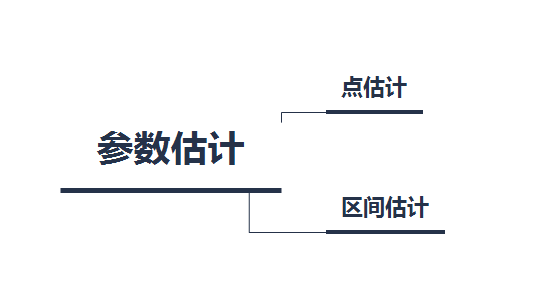点估计：依据样本估计总体分布中所含的未知参数或未知参数的函数。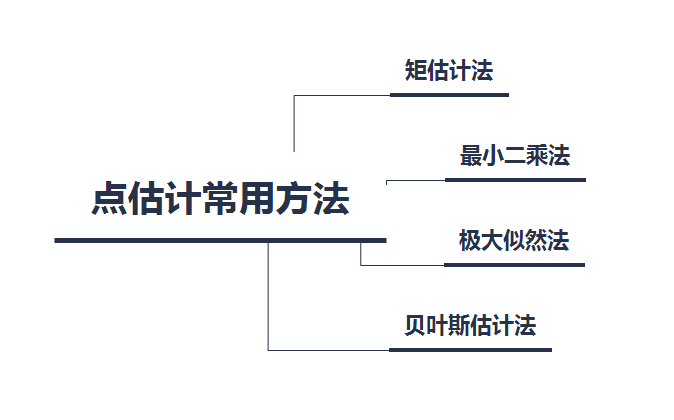区间估计（置信区间的估计）：依据抽取的样本，根据一定的正确度与精确度的要求，构造出适当的区间，作为总体分布的未知参数或参数的函数的真值所在范围的估计。例如人们常说的有百分之多少的把握保证某值在某个范围内，即是区间估计的最简单的应用。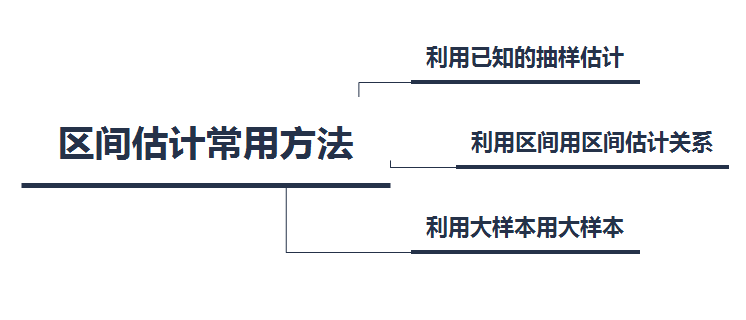本文主要讲述点估计的矩估计法和极大似然法
矩估计法：
矩估计法的理论依据是大数定律。矩估计是基于一种简单的“替换”思想，即用样本矩估计总体矩。
矩的理解：
在数理统计学中有一类数字特征称为矩。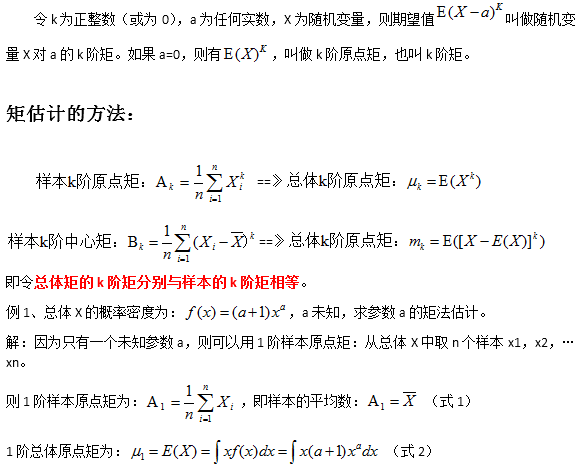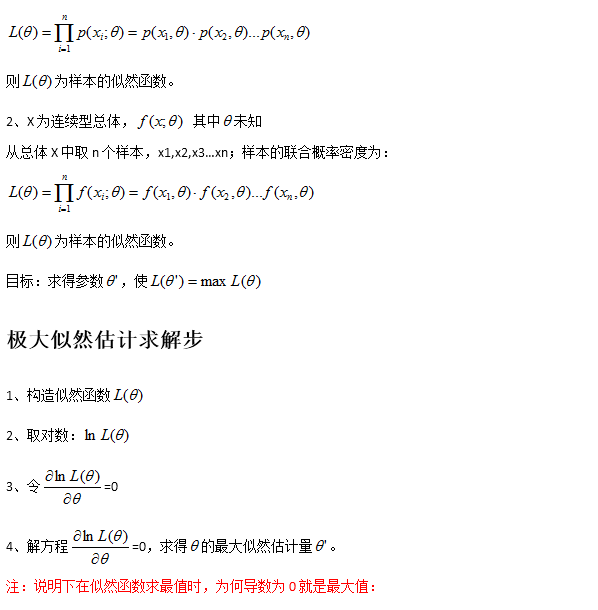首先要明确的是我们求得是函数 的最大值，因为log是单调递增的，加上log后并不影响 的最大值求解。为何导数为0就是最大值：就是我们目前所知的概率分布函数一般属于指数分布族（exponential family），例如正态分布，泊松分布，伯努利分布等。所以大部分情况下这些条件是满足的。但肯定存在那种不符合的情况，只是我们一般比较少遇到。
极大似然估计总结
似然函数直接求导一般不太好求,一般得到似然函数L(θ)之后,都是先求它的对数,即ln L(θ),因为ln函数不会改变L的单调性.然后对ln L(θ)求θ的导数,令这个导数等于0,得到驻点.在这一点,似然函数取到最大值,所以叫最大似然估计法.本质原理嘛,因为似然估计是已知结果去求未知参数,对于已经发生的结果（一般是一系列的样本值）,既然他会发生,说明在未知参数θ的条件下,这个结果发生的可能性很大,所以最大似然估计求的就是使这个结果发生的可能性最大的那个θ.这个有点后验的意思.


展开全文机器学习 算法 人工智能 python 数据分析
• 它是统计推断种基本形式，是数理统计学一个重要分支，分为点估计和区间估计两部分。 点估计：依据样本估计总体分布中所含未知参数或未知参数的函数。 区间估计（置信区间的估计）：依据抽取样本，...
参数估计 参数估计：是根据从总体中抽取的样本估计总体分布中包含的未知参数的方法。它是统计推断的一种基本形式，是数理统计学的一个重要分支，分为点估计和区间估计两部分。点估计：依据样本估计总体分布中所含的未知参数或未知参数的函数。区间估计（置信区间的估计）：依据抽取的样本，根据一定的正确度与精确度的要求，构造出适当的区间，作为总体分布的未知参数或参数的函数的真值所在范围的估计。例如人们常说的有百分之多少的把握保证某值在某个范围内，即是区间估计的最简单的应用。本文主要讲述点估计的矩估计法和极大似然法 矩估计法：
矩估计法的理论依据是大数定律。矩估计是基于一种简单的“替换”思想，即用样本矩估计总体矩。 矩的理解：
在数理统计学中有一类数字特征称为矩。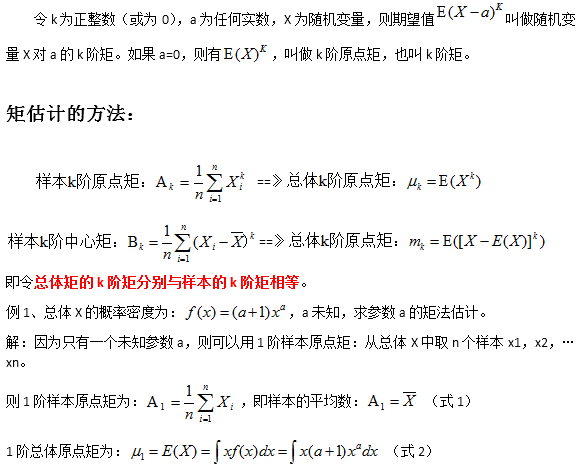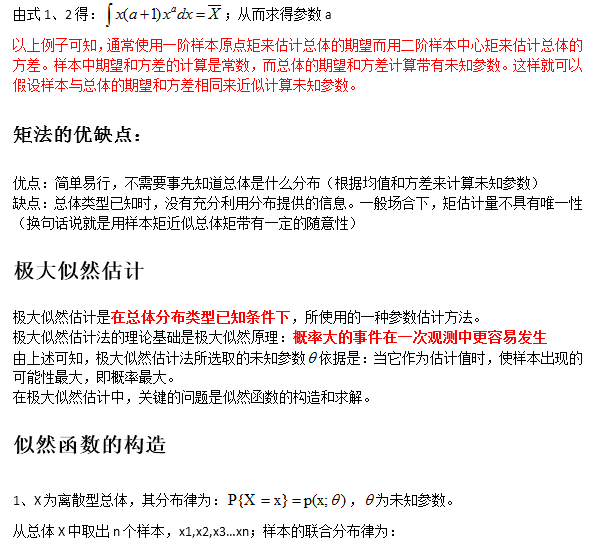首先要明确的是我们求得是函数 的最大值，因为log是单调递增的，加上log后并不影响 的最大值求解。为何导数为0就是最大值：就是我们目前所知的概率分布函数一般属于指数分布族（exponential family），例如正态分布，泊松分布，伯努利分布等。所以大部分情况下这些条件是满足的。但肯定存在那种不符合的情况，只是我们一般比较少遇到。 极大似然估计总结
似然函数直接求导一般不太好求,一般得到似然函数L(θ)之后,都是先求它的对数,即ln L(θ),因为ln函数不会改变L的单调性.然后对ln L(θ)求θ的导数,令这个导数等于0,得到驻点.在这一点,似然函数取到最大值,所以叫最大似然估计法.本质原理嘛,因为似然估计是已知结果去求未知参数,对于已经发生的结果（一般是一系列的样本值）,既然他会发生,说明在未知参数θ的条件下,这个结果发生的可能性很大,所以最大似然估计求的就是使这个结果发生的可能性最大的那个θ.这个有点后验的意思
展开全文统计学 极大似然估计
• 一、最大似然估计与无偏估计回顾如果大家学习过概率统计应该知道最大似然估计和无偏估计两种参数估计的方法，而贝叶斯估计也是一种参数估计的方法，不同之处在于，贝叶斯估计需要先验信息来帮助我们估计。...
• 它是统计推断种基本形式，是数理统计学一个重要分支，分为点估计和区间估计两部分。 点估计：依据样本估计总体分布中所含未知参数或未知参数的函数。 区间估计（置信区间的估计）：依据抽取样本，根据...
• 它是统计推断种基本形式，是数理统计学一个重要分支，分为点估计和区间估计两部分。 点估计：依据样本估计总体分布中所含未知参数或未知参数的函数。 区间估计（置信区间的估计）：依据抽取样本，根据一定...
• 参数估计：是根据从总体中抽取样本估计总体分布中包含未知参数的方法。它是统计推断种基本形式，是数理统计学一个重要分支，分为点估计和区间估计部分。 点估计：依据样本估计总体分布中所含未知...
• 通过研究更好的估计，本文作者基于图克扬混合分布模型发明了一称为PEROBLS D方法，在该模型中，污染率（百分比）和个分布的参数（形成混合分布）都得到了估计，并且与现有方法相比，基本正态分布的参数获得...
• 统计学(第六版)贾俊平 读书笔记 第 7 章 参数估计 7.1 参数估计的基本原理 参数估计就是用样本统计量去估计总体的参数。比如，用样本均值估计...参数估计的方法有点估计和区间估计两种。点估计就是中样本统计量的...数理统计 数据分析 机器学习理论基础
• 参数估计两种基本形式：点估计和区间估计。点估计是用一个数值作为未知参数θ估计值，而区间估计是给出具体上限和下限，把θ包括在这个区间内。点估计，主要有矩估计法和最大似然统计法。矩估计法是用样本矩...
• 掌握参数估计和假设检验这两个数理统计最基本方法，方能有效地对数据进行描述和分析。在本文中，我们对这两种基本方法及它们在MATLAB中运用进行简要介绍。matlab 数学建模 统计学
• 【机器学习基本理论】详解最大后...最大似然估计（Maximum likelihood estimation, 简称MLE）和最大后验概率估计（Maximum a posteriori estimation, 简称MAP）是很常用的两种参数估计方法，如果不理解这两种方法的...MAP
• 然后，对于正态分布位置参数，分为方差未知和已知两种情况，分别建立了基于t分布验前P值计算模型，和基于正态分布验前P值计算模型，并给出了不同验前可信度取值范围下验前分布选择与判断准则.其次，对于正态...
• 针对传统马尔可夫模型（HMM）状态停留时间必须服从指数分布假设的不足，提出了一基于隐半马尔可夫模型（HSMM）的两阶段设备缺陷状态识别方法。首先，通过分析HSMM模型的参数构成及基本特点，并结合两阶段设备的劣...延迟时间
• 经过特征点和相应摄像机光心的两条空间直线的方程，两条直线的交点坐标 即为特征点的三维世界坐标。考虑到由于实际计算时存在各种误差，两条直 线很有可能并不相交，因此利用最小二乘法对以上的方程组进行求解。最 ...图像处理
• 在本文中，我们开发了一基于核密度估计的框架，无需任何先验知识即可对海杂波分布进行建模。 在此框架中，我们共同考虑了个嵌入的基本问题，即选择合适的内核密度函数和确定其相应的最佳带宽。 针对这个问题...研究论文
• 最大似然估计的基本思想 　最大似然估计思想很简单：在已经得到试验结果情况下，我们应该寻找使这个结果出现 可能性最大那个 作为真 的估计。 　我们分两种情进行分析： 　1．离散型总体　 　设 为...
• 前段日子在一个视频上看到了java中...直接说两种说法都是对，只是理解不一样，当时我也以为是这样，不过后来为了确定这个答案，特地去翻阅了《java核心技术》中文翻译版，基本得出一个答案。  java程序设计语值传递 值传递和引用传递 引用传递
• 2．掌握在Matlab平台上进行信号功率谱密度估计的基本方法 二、实验原理 1.随机信号功率谱密度定义 定义随机信号信号的功率谱为 其中为随机信号的自相关函数。 功率谱反映了信号的功率在频域随频率分布，...
• 大学概率论课程都有讲到参数估计的两种基本方法：极大似然估计、矩估计。两种方法都是利用样本信息尽量准确的去描述总体信息，或者说给定模型（参数全部或者部分未知）和数据集（样本），让我们去估计模型的未知参数...
• 它是统计推断种基本形式，是数理统计学一个重要分支，分为点估计和区间估计两部分。    点估计：依据样本估计总体分布中所含未知参数或未知参数的函数。    区间估计（置信区间的估计）：依据抽取...
• 理解这两种估计的思想，掌握求参数估计量的方法和评判估计量好坏的标准.点估计问题的提出设灯泡寿命T～N(μ,σ2)T～ N (\mu,\sigma^2)，但参数μ\mu和σ2\sigma^2未知. 现在要求通过对总体抽样得到的样本，构造两...数理统计
• 今天就大概说说点估计的两基本方法和一些它们的比较。一、矩估计矩估计是一十分直观的估计方法，设 是简单随机样本（独立同分布），则可以用样本矩估计总体矩，由此获得一个关于总体分布参数的一个方程组，解之....
• 一、参数估计简介 很多情况下，我们只有有限样本集，而类条件概率密度函数p(x|ωi)和先验概率P(ωi)是未知...有两种主要参数估计法：确定性参数估计方法是把参数看作确定而未知, 典型方法为最大似然估计。随机参数机器学习 人工智能 数据分析
• 在有足够的适应性数据时，基于本机的说话人适应优于传统的最大似然线性回归（MLLR）和本征语音方法。... 还研究了这些方法的两种组合，即弹性网（同时应用l1和平方的l2范数）和稀疏组套索（同时应用l1和列式非平方的l2研究论文...